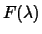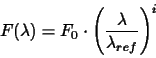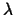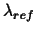Next: Continuum Up: The input flux wavelength Previous: Template Spectra

## Power Law

The Power Law input flux distribution is another useful case present in the UVES ETC. In this case the input flux distributionis defined as:(2.4)

whereis the wavelength at which the flux is detected,is the reference wavelength of the power law, i is the power law index number, in general a real number, and the reference flux F0 is given by the (eq. 2.5).

Pascal Ballester
1999-07-29